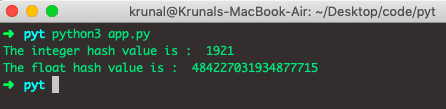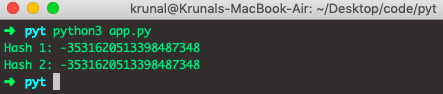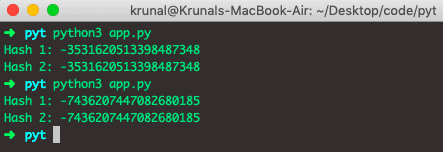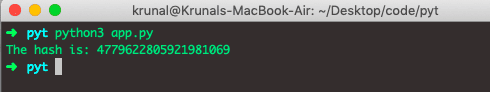# Python hash: How to Create Hash Value in Python

A good hash function is the one function that results in the least number of collisions, meaning, No two sets of information should have the same hash values.

## Python hash()

Python hash() is a built-in method that returns a hash value of the object if it has one. Hash values are just integers, which are used to compare the dictionary keys during a dictionary lookup quickly. In simple terms, the hash is a fixed size integer that identifies the particular value. Please note that the above definition is the simplest explanation.

Let us point out what is a fixed hash can mean? See the following points.

1. The same data will have the same hash value.
2. Even a slight change in the original data can result in a completely different hash value.
3. The hash is obtained from a hash function, whose responsibility is to convert a piece of given information to the encoded hash.
4. Several objects can be much more than several hash values, and so, two objects may hash to the same hash value. It is called a Hash collision. This means that if two objects have the same hash code, they do not necessarily have the same value.
5. Objects hashed using hash() are irreversible, leading to loss of information.
6. The hash() method returns hashed values only for immutable objects. Hence it can be used as an indicator to check for mutable/immutable objects.

Internally, the hash() method calls __hash__() method of the object, which is set by default for any object. We’ll look at this later.

Hash codes are the most used when a comparison for the dictionary keys is made.

The Hash code of the dictionary keys is compared when a dictionary lookup is done for the specific key. Comparing hash is much faster than comparing the total key values because of the set of integers that hash function maps each dictionary key which is much smaller than the set of objects itself.

### Syntax

See the below Hash method syntax.

`hash(object)`

### Parameters

The object parameter, whose hash value is to be returned (integer, string, float).

See the below example code.

```# app.py

tuple_value = (21, 19, 18, 46, 29) # tuples are immutable

list_value = [11, 21, 31, 41, 51] # list are mutable

print ("The tuple hash value is : " + str(hash(tuple_value)))
print ("The list hash value is : " + str(hash(list_value)))```

See the below output.A tuple is an immutable object that is we can create the hash value from the tuple.

A list is a mutable object. That is why it throws an error like we can convert the list into hash.

The list is an unhashable type.

## Return value from hash()

A hash() function returns the hash value of the object if it has one. If the object has the custom __hash__() method, it truncates the return value to a size of Py_ssize_t.

## How hash() works in Python

See the following hash example in python.

```# app.py

value = 1921
print("The integer hash value is : ", hash(value))

valueA = 19.21
print("The float hash value is : ", hash(valueA))```

In the above code, we have taken two data types.

1. Integer
2. Float

Now, the hash of the integer is the integer itself, but the hash of the float number is somewhat different. For example, see the below output.## Python hash() String

Let us start constructing simple examples and scenarios in which the hash() method can be beneficial.

In this example, we will get the hash value of a String.

```# app.py

app = "Instagram"

hash1 = hash(app)
hash2 = hash(app)

print("Hash 1: %s" % hash1)
print("Hash 2: %s" % hash2)```

See the below output.Both outputs are the same because we have used the same string for the hash.

Here is a significant catch if you rerun the same script, the hash changes, as shown below.## How to define a hash() function for custom objects

The hash() function works by overriding the __hash()__ function.

It’s worth noticing that not every object is hashable (mutable collections aren’t hashable).

We can also define the __hash__() function for our custom class. So that’s what we will do now. But, before that, let’s point out some essential points.

See the following example.

1. Hashable implementation should not be done for the mutable collections, as keys of the collections should be immutable for hashing.
2. We don’t have to define the custom __eq()__ function implementation as it is defined for all objects.

See the following code example.

```# app.py

class Employee:
def __init__(self, empno, name):
self.empno = empno
self.name = name

def __eq__(self, other):
return self.empno == other.empno and self.name == other.name

def __hash__(self):
return hash((self.empno, self.name))

employee = Employee(21, 'Krunal')
print("The hash is: %d" % hash(employee))
```

See the following output.Cases for custom hash implementation for objects.

Hash implementation of Objects
__eq__() __hash__() Description
Defined (by default) Defined (by default) If left as is, all objects compare unequal (except themselves)
(If mutable) Defined Should not be defined Implementation of hashable collection requires the key’s hash value to be immutable.
Not defined Should not be defined If __eq__() isn’t defined, __hash__() should not be defined.
Defined Not defined Class instances will not be usable as a hashable collection.

__hash__() implicitly set to None

Raises TypeError exception if tried to retrieve the hash.

Defined Retain from Parent __hash__ = <ParentClass>.__hash__
Defined Doesn’t want to hash __hash__ = None

Raises TypeError exception if tried to retrieve the hash.

## Why a mutable object cannot be Hashed

As we already know, only an immutable object can be hashed. This restriction of not allowing the mutable object to be hashed simplifies the hash table a lot. Let’s understand how.

If the mutable object is allowed to be hashed, we need to update a hash table every time a value of the object updates. What this means is that we will have to move the object to a completely different bucket. It is a very costly operation to be performed.

In Python, we have two objects that use hash tables, dictionaries, and sets:

1. A dictionary is the hash table and is called the associative array. In the dictionary, only keys are hashed and not the values. This is why the dictionary key should be the immutable object as well, while values can be anything, even the list which is mutable.
2. The set contains unique objects which are hashable. If we have the non-hashable items, we cannot use the set and instead use a list.

That’s it for this tutorial.

### 1 thought on “Python hash: How to Create Hash Value in Python”

1.how does it calculate the hash of an object?